/
/
/
MULTIPLE CHOICE 1.Find the general solution of the differential equation below
Not my Question
Flag Content

# Question : MULTIPLE CHOICE 1.Find the general solution of the differential equation below : 2082869

MULTIPLE CHOICE

1.Find the general solution of the differential equation below and check the result by differentiation.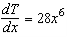a.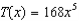b.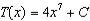c.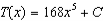d.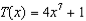e.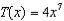2.Find the general solution of the differential equation below and check the result by differentiation.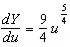a.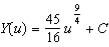b.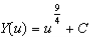c.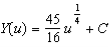d.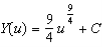e.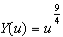3.Find the indefinite integral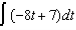.

 a.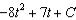b.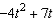c.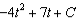d.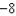e. none of the above

4.Find the indefinite integral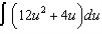.

 a.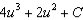b.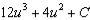c.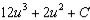d.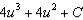e.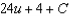5.Find the indefinite integral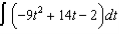.

 a.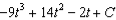b.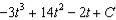c.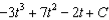d.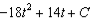e.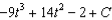## Solution 5 (1 Ratings )

Solved
Calculus 3 Days Ago 54 Views